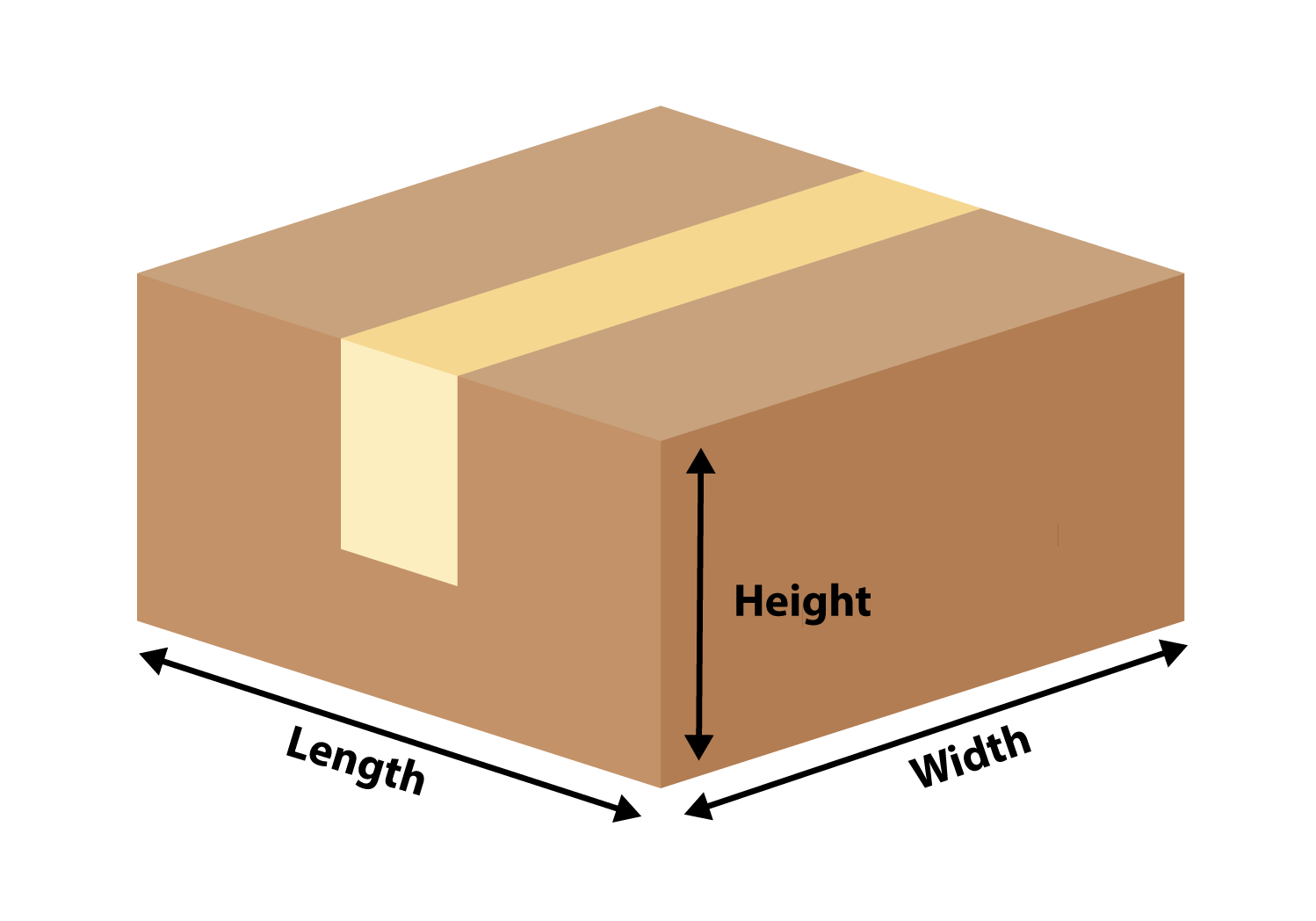# How is shipment weight calculated?

A shipment weight is determined by the greater of the following two measurements:

• Actual Weight
• Dimensional Weight (aka - Volumetric Weight)

Actual weight is taking the total parcel weight (items, packaging, and box weight).

Dimensional (DIM) weight is how carriers calculate the dimensional factor (volumetric weight) of what something should weigh versus the actual weight. Many Carriers calculate dimensional weight based on the formula of multiplying the L x H x W of a package (measured in inches), then dividing by 139 (numbers in tenths, .2 or .5, are rounded up the nearest whole number)

Important Note: FlavorCloud charges the merchant the weight determined at fulfillment, and not at checkout.  This accounts for if there are potential errors with SKU weights in the ecommerce system, different packaging used at time of fulfillment, etc.,

FlavorCloud utilizes the greater of the weights at fulfillment; actual weight or DIM weight